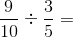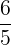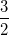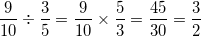ISEE Middle Level Math : How to divide fractions

Example Questions

Example Question #11 : How To Divide Fractions

Simplify: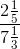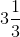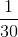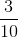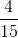Explanation:

Rewrite as a division, then solve: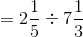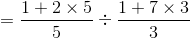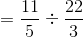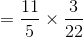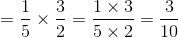Example Question #12 : How To Divide Fractions

Evaluate: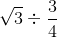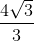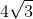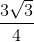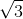Explanation:

To divide any number by a fraction, we can multiply that number by the reciprocal of the fraction. That means: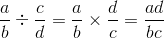So we have: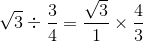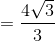Example Question #13 : How To Divide Fractions

Simplify: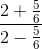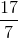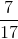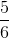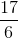Explanation:

Multiply each term by 6: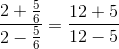Example Question #14 : How To Divide Fractions

Evaluate: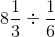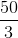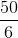Explanation:

A mixed number represents the sum of an integer and a fraction. In order to evaluate this problem first we need to change the mixed number (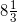) to an improper fraction. Improper fractions are fractions whose numerator is greater than the denominator. So we can write: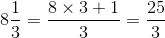Now we should evaluate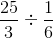and we have: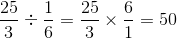Example Question #15 : How To Divide Fractions

Simplify: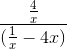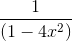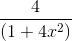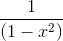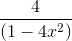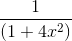Explanation:

We can first find a common denominator for the expression in the numerator, which is. This gives us: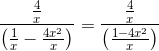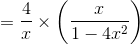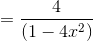Example Question #531 : Numbers And Operations

Which of the following is the reciprocal of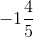?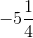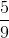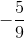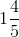Explanation:

First, rewrite this as an improper fraction: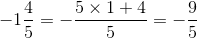The reciprocal of an improper fraction can be found by switching its numerator and denominator, retaining the negative sign, so the reciprocal is.

Example Question #532 : Numbers And Operations

Which of the following is the reciprocal of 2.8?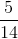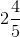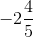Explanation:

First, rewrite this as an improper fraction: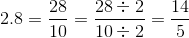The reciprocal of an improper fraction can be found by switching its numerator and denominator, so the reciprocal is.

Example Question #533 : Numbers And Operations

Solve: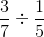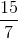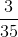Explanation: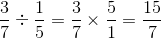Example Question #534 : Numbers And Operations

Which of the following is the reciprocal of 31.25?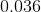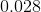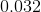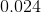Explanation:

Rewrite 31.25 as a fraction: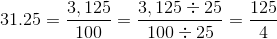Exchange the positions of the numerator and the denominator to get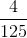. Now divide 4 by 125: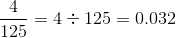Example Question #535 : Numbers And Operations

Solve: